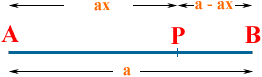Quandaries and Queries My name is James. I'm having a difficult time with a pre calculus problem, which goes as follows: "show that AB/AP=AP/PB is equal to (1+51/2)/2 (one plus the sqaure root of five with the sum divided by two. I can't do the square root sign, sorry.) I have tried to solve for PB in terms of the other varialbles and then work the quadratic equation. THAT DOES NOT WORK!!!! I am befuddled. Please help me. I am a student of secondary level. Hi James, I see this as a line segment from a point A to a point B with P on the segment between A and B, closer to B than to A.Let a be the length of the line segment AB. The length of the segment AP is a fraction of the length of AB. Let this fraction be x so the length of AP is ax. Thus the length of PB is a - ax. Thus your equation  AB/AP = AP/PB is  a/ax = ax/a - ax Solve for x. Penny Go to Math Central